# Perception¶

## Description¶

The perception module enables chess moves to be recognised using machine vision. It is based on OpenCV and runs on Python 2.7. An Asus Xtion camera provides frames as an input, which are then processed by the perception engine. It outputs a Black White Empty (BWE) matrix that is then passed on to the chess engine. This matrix is returned as a nested list, filled with ‘E’ for empty chess squares, ‘W’ if the square is occupied by a white piece, and ‘B’ if it’s occupied by a black piece. As the initial setup of the chess pieces is constant, this matrix is sufficient to determine the state of the game at any time.

## Design¶

There are several classes such as Line, Square, Board and Perception within the pereption module. The code works in the following sequence:

1) A picture of an empty board is taken and its grid is determined. 64 Square instances are generated, each holding information about the position of the square, its current state (at this stage they are all empty), and color properties of the square. The 64 squares are stored in a Board instance, holding all the information about the current state of the game. The Board instance is stored in a Perception instance, representing the perception engine in its entirety and facilitating access from other modules.

2) The chessboard is populated by the user in the usual setup. The initial BWE matrix is assigned, looking like this:

`B B B B B B B B`
`B B B B B B B B`
`E E E E E E E E`
`E E E E E E E E`
`E E E E E E E E`
`E E E E E E E E`
`W W W W W W W W`
`W W W W W W W W`

3) When the user has made his or her move, a keyboard key is pressed. This triggers a new picture to be taken and compared to the previous one. The squares that have changed (i.e. a piece has been moved from or to) are analysed in terms of their RGB colors and assigned a new state based thereupon. The BWE matrix is updated and passed to the chess engine, for instance to:

`B B B B B B B B`
`B B B B B B B B`
`E E E E E E E E`
`E E E E E E E E`
`E E E E W E E E`
`E E E E E E E E`
`W W W W E W W W`
`W W W W W W W W`

4) The chess engine determines the best move to make and the robot executes it. The user then needs to press the keyboard again to update the BWE to include the opponent’s (robot) move. Upon pressing a key, the BWE might look like this:

`B B B B B B B B`
`B B B B E B B B`
`E E E E E E E E`
`E E E E B E E E`
`E E E E W E E E`
`E E E E E E E E`
`W W W W E W W W`
`W W W W W W W W`

5) Return to step 3. The loop continues until the game ends.

## Machine Vision¶

This section is concerned with how the machine vision works that achieves perception.

The first stage of image analysis takes care of thresholding, filtering and masking the chessboard. Adaptive thresholding is used to subsequently do contour detection, concerned with detecting the board edges.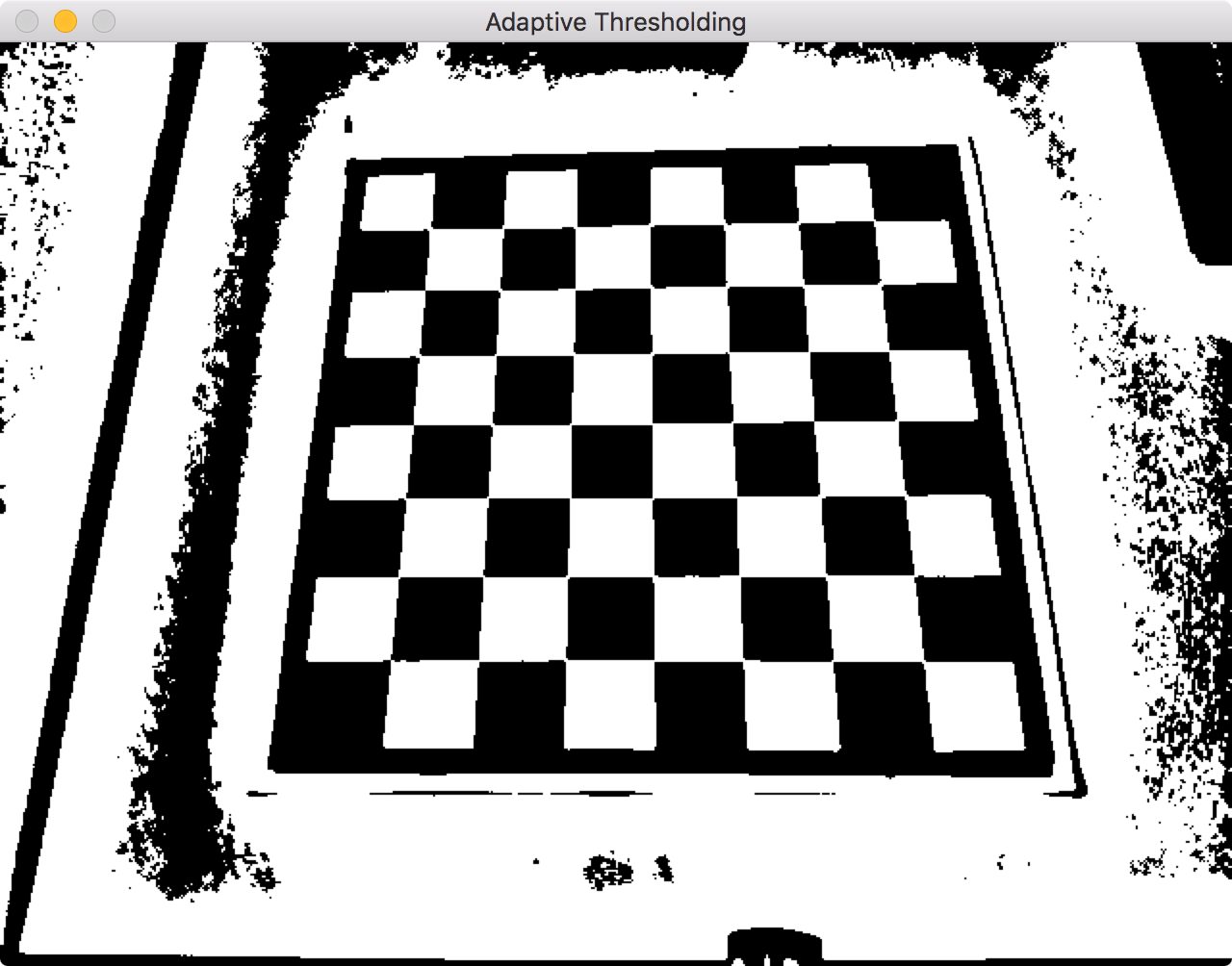A filter looks for squares within the image and filters the largest one, the chessboard. This was achieved by looking at the largest contour within the image that had a ratio of area to perimeter typical for a square.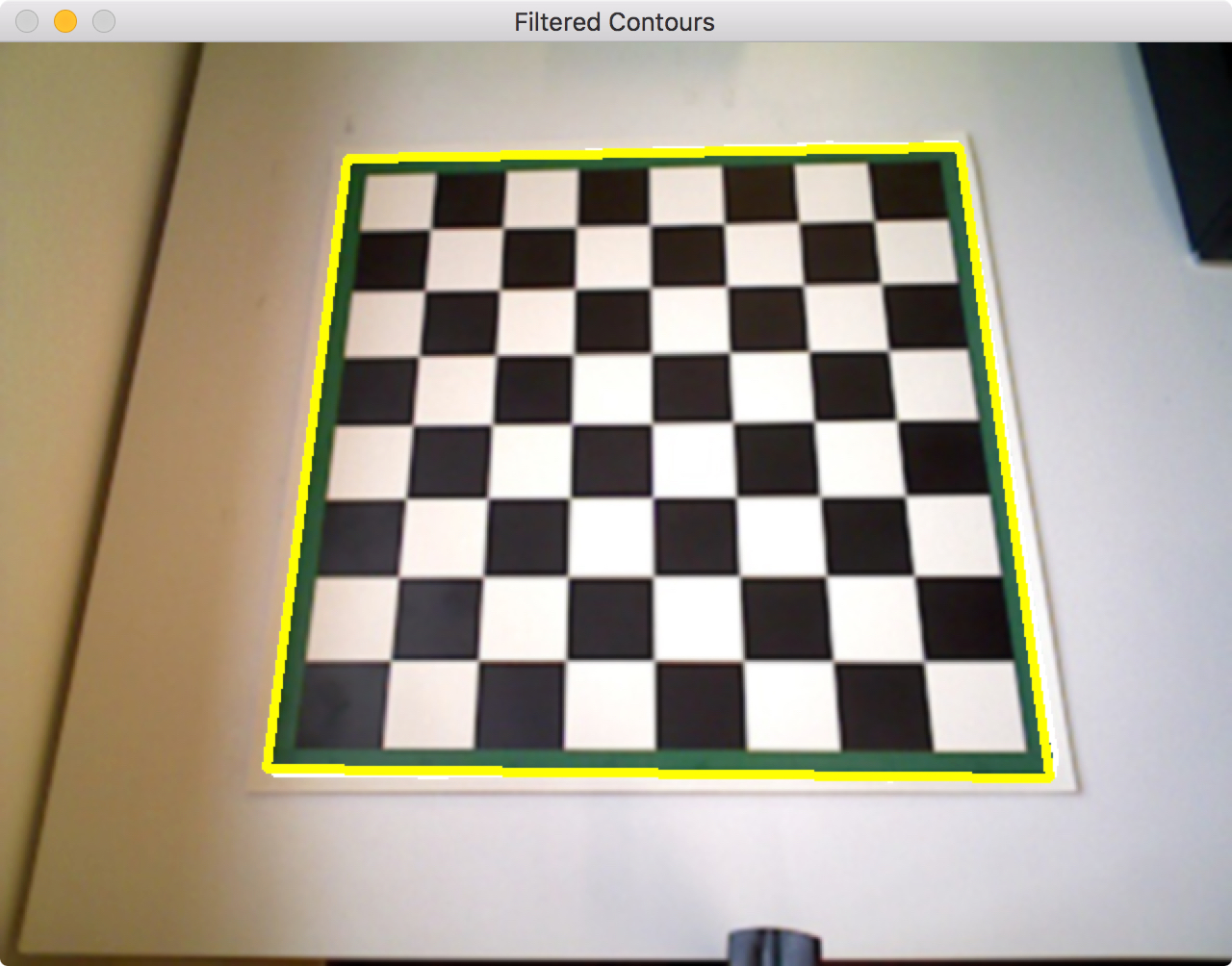The chessboard is masked and the rest of the image is replaced with a homogeneous color. We chose red for this purpose, as it was a color which did not interfere with other colors in the image.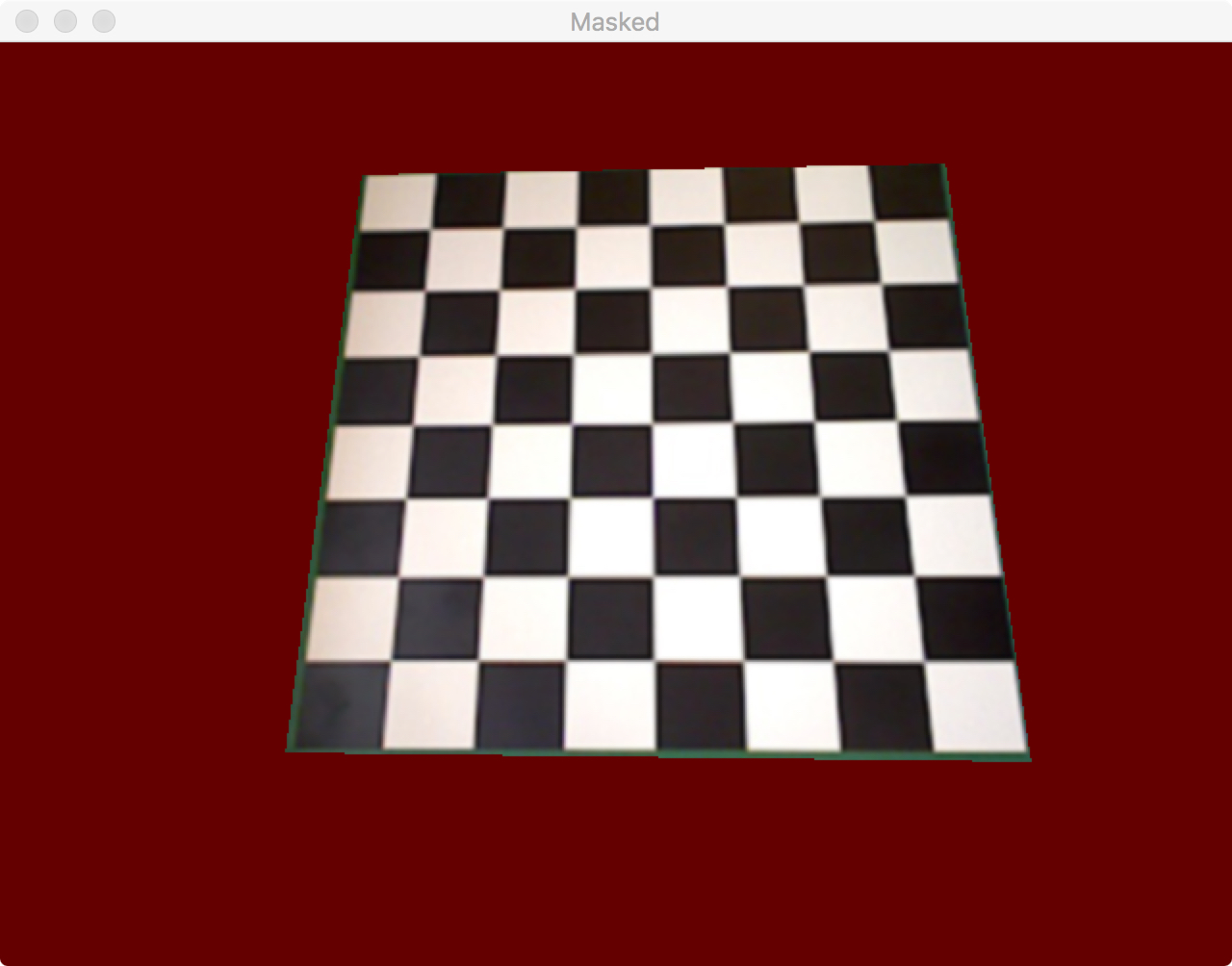### Determining the chess corners and squares¶

Canny edge detection is needed to determine Hough lines. It is an algorithm consisting of various stages of filtering, intensity gradient calculations, thresholding and suppression to identify edges. As shown below, it aids with identifying the chess grid.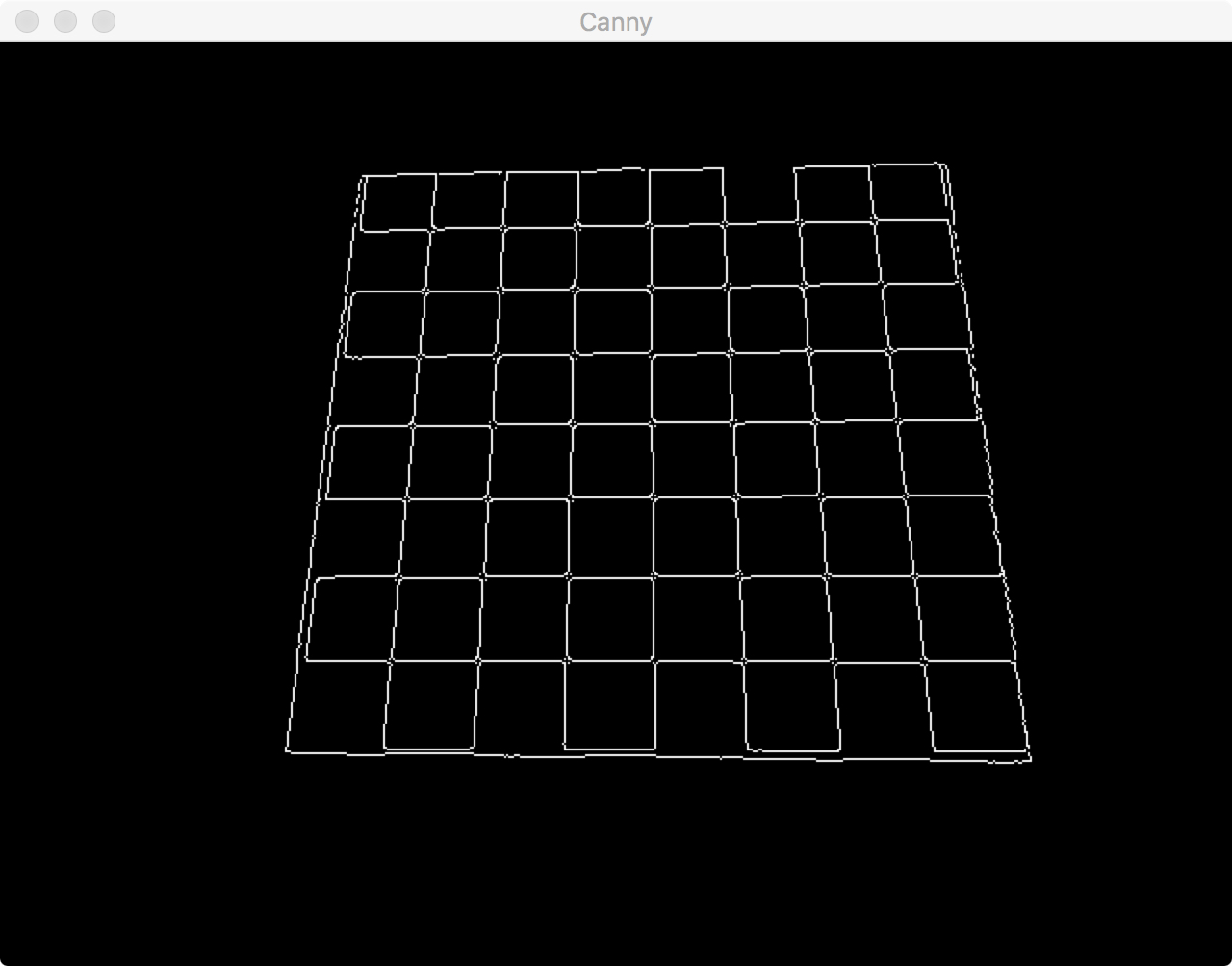Hough lines are subsequently calculated from the Canny image. Lines are identified and instantiated with their gradients and positions. At that stage, similar lines are sometimes clustered together, so gradient filtering is applied to minimise the number of lines without losing the ones needed.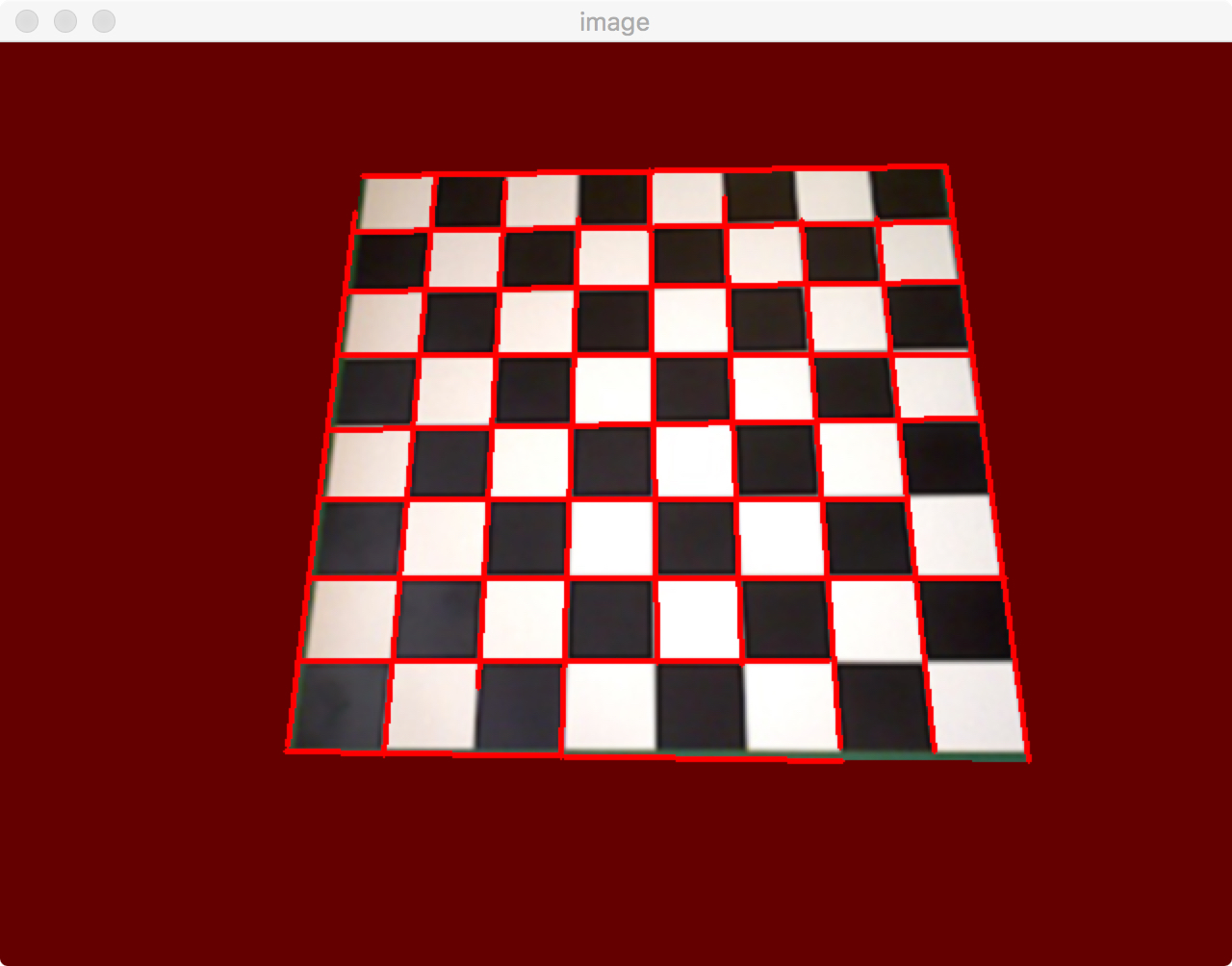Intersections of Hough lines are found by equating two lines and solving. As there are still some duplicates, an algorithm now does the final filtering to ensure that only 81 points remain. The corner points (9 x 9) are assigned to rows and columns within the chessboard. 64 (8 x 8) Square instances are then generated. Each holds information about its position, index, and color average within the ROI area (shown as a circle in its centre). When the game is setup, the latter is the square’s ‘empty color’, i.e. black or white.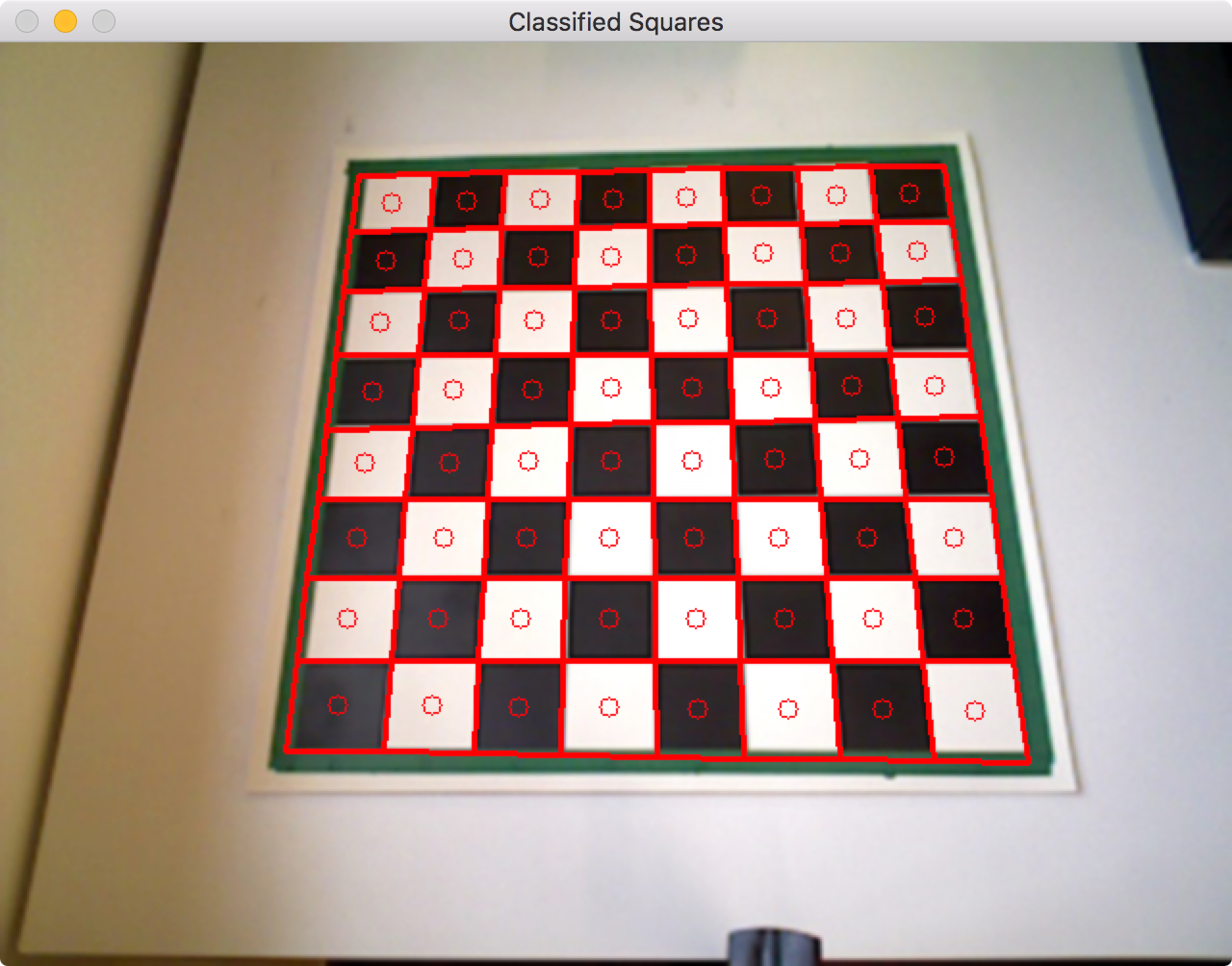```class Square:
"""
Class holding the position, index, corners, empty colour and state of a chess square
"""
def __init__(self, position, c1, c2, c3, c4, index, image, state=''):
# ID
self.position = position
self.index = index
# Corners
self.c1 = c1
self.c2 = c2
self.c3 = c3
self.c4 = c4
# State
self.state = state

# Actual polygon as a numpy array of corners
self.contours = np.array([c1, c2, c3, c4], dtype=np.int32)

# Properties of the contour
self.area = cv2.contourArea(self.contours)
self.perimeter = cv2.arcLength(self.contours, True)

M = cv2.moments(self.contours)
cx = int(M['m10'] / M['m00'])
cy = int(M['m01'] / M['m00'])

# ROI is the small circle within the square on which we will do the averaging
self.roi = (cx, cy)

# Empty color. The colour the square has when it's not occupied, i.e. shade of black or white. By storing these
# at the beginnig of the game, we can then make much more robust predictions on how the state of the board has
# changed.
self.emptyColor = self.roiColor(image)

```

The board can now be instantiated as a collection of all the squares. The sequence of functions called to generate the board is called within the makeBoard function, shown below:

```    def makeBoard(self, image, depthImage):
"""
Takes an image of an empty board and takes care of image processing and subdividing it into 64 squares
which are then stored in one Board object that is returned. Expanding to depth calibration has not yet been
finished.
"""
try:
# Process Image: convert to B/w
image, processedImage = self.processFile(image)
except Exception as e:
print(e)
print("There is a problem with the image...")
print("")
print("The image print is:")
print(image)
print("")

# Extract chessboard from image
extractedImage = self.imageAnalysis(image, processedImage, debug=False)

# Chessboard Corners
cornersImage = extractedImage.copy()

# Canny edge detection - find key outlines
cannyImage = self.cannyEdgeDetection(extractedImage)

# Hough line detection to find rho & theta of any lines
h, v = self.houghLines(cannyImage, extractedImage, debug=False)

# Find intersection points from Hough lines and filter them
intersections = self.findIntersections(h, v, extractedImage, debug=False)

corners, cornerImage = self.assignIntersections(extractedImage, intersections, debug=False)

# Copy original image to display on
squareImage = image.copy()

# Get list of Square class instances
squares = self.makeSquares(corners, depthImage, squareImage, debug=False)

# Make a Board class from all the squares to hold information
self.board = Board(squares)

# Assign the initial BWE Matrix to the squares
self.board.assignBWE()
```

### Updating the BWE matrix¶

When a piece is moved, the code detects changes between the previous and the current image. The centres of the bounding boxes surrounding that change region are matched with the squares. Two squares will be detected to have changed, as the centres of the change regions lie within them. A piece has been either moved from or to that square.

Both squares current Region of Interest (ROI) colors are taken and compared against their ‘empty colors’, i.e. their colors when not occupied by a piece. This distance is quantified by a 3-dimensional RGB color distance. The one with the smaller distance to its empty state must currently be an empty square, meaning a piece has been moved from it. Its old state (when the piece still was there) is saved temporarily, while its state is reassigned as empty. The non-empty square now takes the state of the piece that has been moved to it, i.e. the empty square’s old state.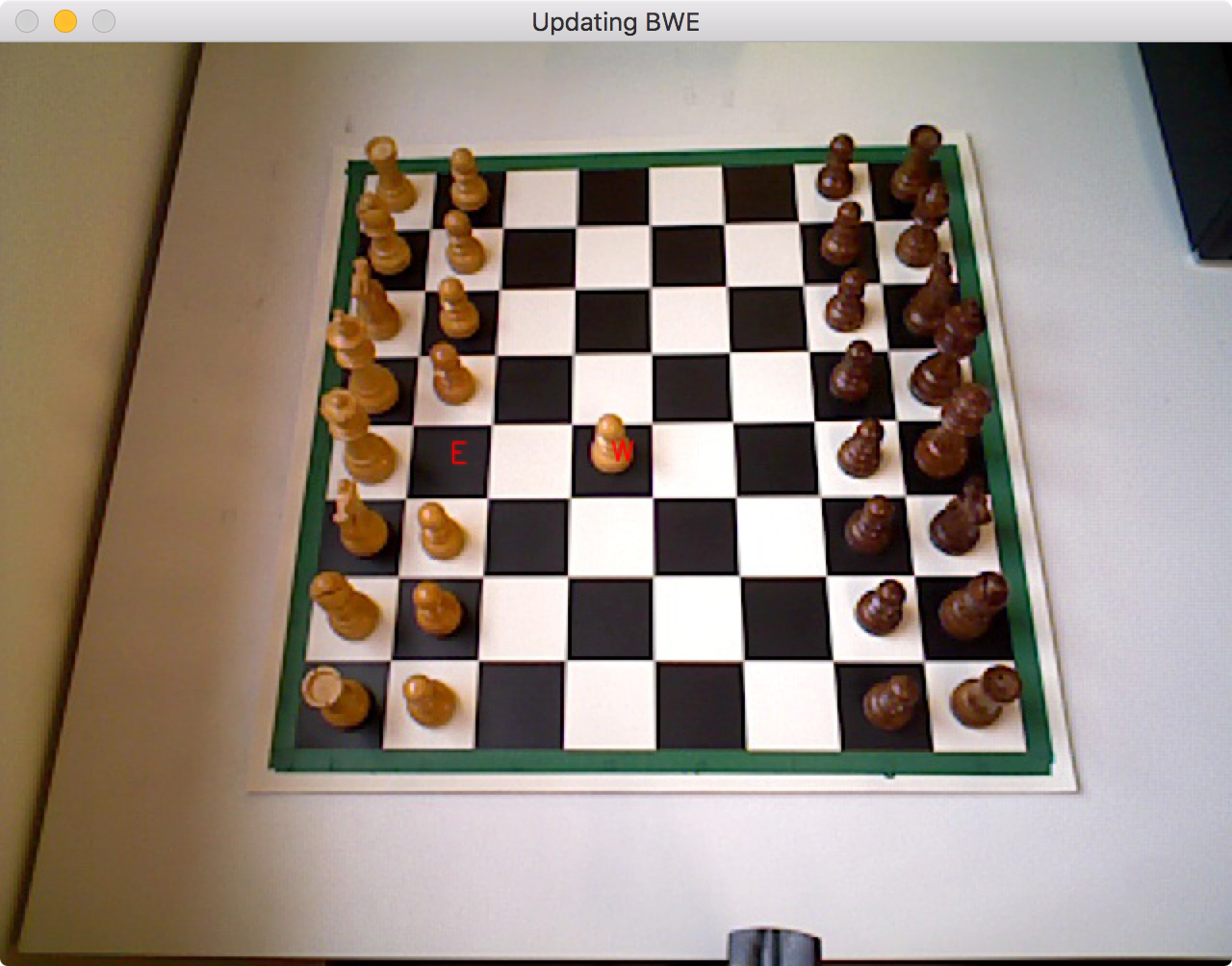```    def updateBWE(self, matches, current):
"""
Updates the BWE by looking at the two squares that have changed and determining which one is now empty. This
relies on calculated the distance in RGB space provided by the classify function. The one with a lower distance
to the colour of its empty square must now be empty and its old state can be assigned to the other square that
has changed.
"""

# Calculates distances to empty colors of squares
distance_one = matches.classify(current)
distance_two = matches.classify(current)

if distance_one < distance_two:
# Store old state
old = matches.state
# Assign new state
matches.state = 'E'
self.BWEmatrix[matches.index] = matches.state
# Replace state of other square with the previous one of the currently white one
matches.state = old
self.BWEmatrix[matches.index] = matches.state

else:
# Store old state
old = matches.state
# Assign new state
matches.state = 'E'
self.BWEmatrix[matches.index] = matches.state
# Replace state of other square with the previous one of the currently white one
matches.state = old
self.BWEmatrix[matches.index] = matches.state
```

## Limitations¶

This perception module has limitations, which are mostly in terms of robustness and setup. With further development it should be able to recognise the chessboard grid even if it is populated. Changing light conditions make the perception engine very unstable, as the classification of states of chess squares relies on a constant light setting. There are still many improvements that can be made in terms of integrating the perception engine with the chess engine and the motion generation. There are inconsistencies with storing the BWE as a numpy array or as a nested list. Finally, this perception engine relies on having an image of the empty board first.

## Implementation¶

Documentation:

class `perception.mainDetect.``Perception`(board=0, previous=0)[source]

The perception class contains a Board instance as well as functions needed to generate it and output a BWE matrix. The updating of the BWE is done within the Board class.

`assignIntersections`(image, intersections, debug=True)[source]

Takes the filtered intersections and assigns them to a list containing nine sorted lists, each one representing one row of sorted corners. The first list for instance contains the nine corners of the first row sorted in an ascending fashion. This function necessitates that the chessboard’s horizontal lines are exactly horizontal on the camera image, for the purposes of row assignment.

`bwe`(current, debug=False)[source]

Takes care of taking the camera picture, comparing it to the previous one, updating the BWE and returning it.

`cannyEdgeDetection`(image, debug=False)[source]

Runs Canny edge detection

`categoriseLines`(lines, debug=False)[source]

Sorts the lines into horizontal & Vertical. Then sorts the lines based on their respective centers (x for vertical, y for horizontal).

`detectSquareChange`(previous, current, debug=True)[source]

Take a previous and a current image and returns the squares where a change happened, i.e. a figure has been moved from or to.

`drawLines`(image, lines, color=(0, 0, 255), thickness=2)[source]

Draws lines. This function was used to debug Hough Lines generation.

`findIntersections`(horizontals, verticals, image, debug=True)[source]

Finds intersections between Hough lines and filters out close points. The filter relies on a computationally expensive for loop and could definitely be improved.

`houghLines`(edges, image, debug=True)[source]

Detects Hough lines

`imageAnalysis`(img, processedImage, debug=False)[source]

Finds the contours in the chessboard, filters the largest one (the chessboard) and masks it.

`initialImage`(initial)[source]

This function sets the previous variable to the initial populated board. This function is deprecated.

`makeBoard`(image, depthImage)[source]

Takes an image of an empty board and takes care of image processing and subdividing it into 64 squares which are then stored in one Board object that is returned. Expanding to depth calibration has not yet been finished.

`makeSquares`(corners, depthImage, image, debug=True)[source]

Instantiates the 64 squares given 81 corner points.

`printBwe`(bwe)[source]

Prints the BWE.

`processFile`(img, debug=False)[source]

Converts input image to grayscale & applies adaptive thresholding.

`showImage`(image, name='image')[source]

Shows the image

class `perception.boardClass.``Board`(squares, BWEmatrix=[], leah='noob coder')[source]

Holds all the Square instances and the BWE matrix.

`assignBWE`()[source]

Assigns initial setup states to squares and initialises the BWE matrix.

`draw`(image)[source]

Draws the board and classifies the squares (draws the square state on the image).

`getBWE`()[source]

Converts BWE from list of strings to a rotated numpy array

`updateBWE`(matches, current)[source]

Updates the BWE by looking at the two squares that have changed and determining which one is now empty. This relies on calculated the distance in RGB space provided by the classify function. The one with a lower distance to the colour of its empty square must now be empty and its old state can be assigned to the other square that has changed.

`whichSquares`(points)[source]

Returns the squares which a list of points lie within. This function is needed to filter out changes in the images that are compared which have nothing to do with the game, e.g. an arm.

class `perception.squareClass.``Square`(position, c1, c2, c3, c4, index, image, state='')[source]

Class holding the position, index, corners, empty colour and state of a chess square

`classify`(image, drawParam=False, debug=False)[source]

Returns the RGB 3-dimensional distance from a squares current color to its empty color.

`draw`(image, color=(0, 0, 255), thickness=2)[source]

Draws the square onto an image.

`getDepth`(depthImage)[source]
`roiColor`(image)[source]

Finds the averaged color within the ROI within the square. The ROI is a circle with radius r from the centre of the square.

class `perception.lineClass.``Line`(x1, y1, x2, y2)[source]
`draw`(image, color=(0, 0, 255), thickness=2)[source]

Draws line onto an image.

`intersect`(other)[source]

Finds intersections points between two lines.

`perception.lineClass.``filterClose`(lines, horizontal=True, threshold=40)[source]

Filters close lines.